English

# How To: Convert slopes with a certain range of slope degree from a DEM file to a polygon feature class in ArcGIS Pro

## Summary

In ArcGIS Pro, DEM files can be used to determine the area covered by slopes with a certain range of slope degrees by converting the DEM file to a polygon feature class. The converted polygon feature layer contains the area information which can be used for land development.

For instructions on how to do this in ArcMap, see: How To: Convert slopes with a certain range of slope degree from a DEM file to a polygon feature class in ArcMap

## Procedure

These instructions describe how to convert slopes with a certain range of slope degree from a DEM file to a polygon feature class. In this example, slopes with a slope degree of more than 10 degrees are converted to a polygon feature class.

1. In ArcGIS Pro, use the Extract by Mask tool to extract the desired region of the study area in the DEM file. For more information, refer to ArcGIS Pro Web Help: Extract by Mask.
2. Use the Slope tool from Spatial Analyst Tools > Surface to create the slopes from each cell of the DEM. For more information, refer to ArcGIS Pro Web Help: Slope.
3. Change the symbology of the slope map to classify symbology according to the desired range value of the slope degree.
1. Right-click the layer created from the Slope tool and click Symbology.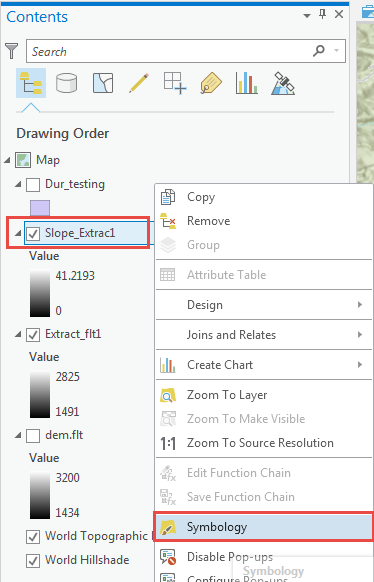2. In the Symbolgy pane, set Primary symbology drop-down to Classify.
3. Set the number of Classes and Method to Manual Interval.
4. Set the Class breaks by changing the Upper value manually. In this example, the classes are divided into 2 and the Upper value is set to (0 - 10) and (>10) respectively.
```Note:
Set class ranges and class breaks according to the data and the range required. In this example, the value (≤ 10) represents slopes with the slope degree range of 0 to 10, while the value (≤ 41.2193412...) represents slopes with the slope degree range more than 10 degrees to 41.22 (the maximum value). For more information, refer to ArcGIS Pro Web Help: Reclass by ranges of values.```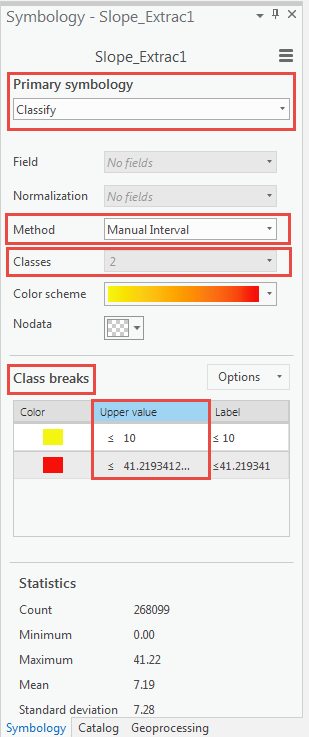1. Use the Reclassify tool from the Spatial Analyst toolbox to reclassify the range values set for the classified DEM file in Step 3.
1. In the Reclassify pane, set the layer in Step 3 as the input raster.
2. Press the Delete key to delete the NODATA values in the Reclassification table.
```Note:
The new value of 1 represents slopes with the slope degree range of 0 to 10 while new value of 2 represents slopes with the slope degree range of 10 - 41.219341.```
1. Set the output raster and click Run.1. Use the Raster to Polygon tool from the Conversion toolbox to convert the raster data to a polygon feature class.
1. Open the attribute table of the new layer created in Step 4 and highlight the row to convert to a polygon feature class. For example, to convert the areas with the range of more than 10 degrees, highlight the row with Value 2.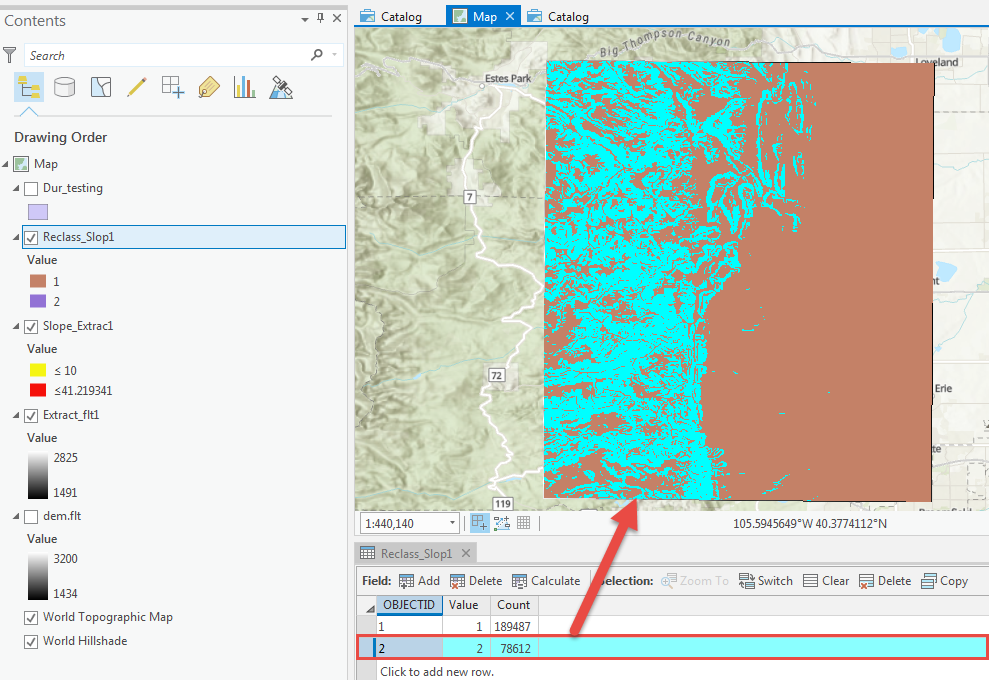2. In the Raster to Polygon pane, set the layer created in Step 4 as Input raster, and set Output polygon features. Click Run.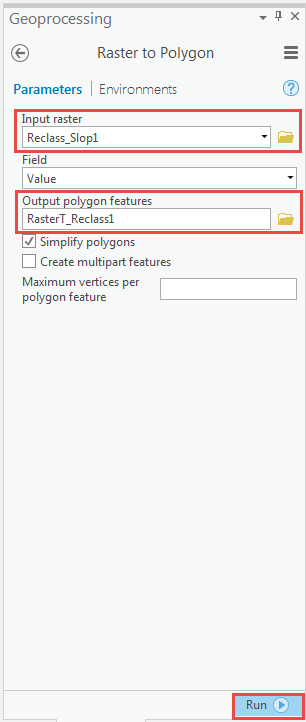A new polygon layer containing the areas of slopes with the slope degree of more than 10 degrees from the Value 2 is displayed in the map.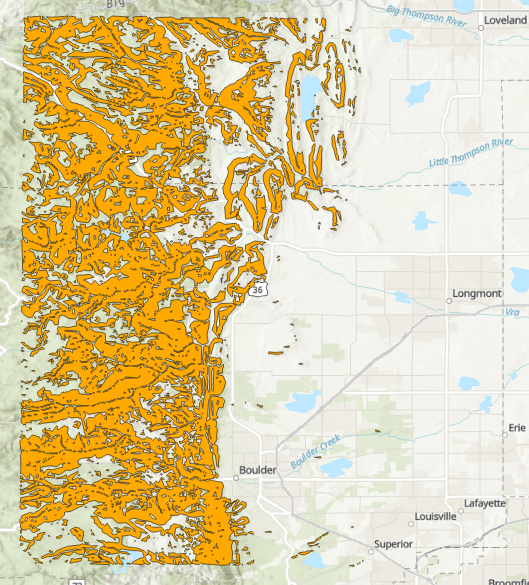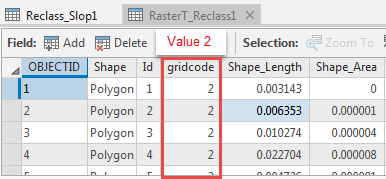## Related Information

Last Published: 1/2/2019

Article ID: 000019549

Software: ArcGIS Pro 2.1.3, 2.1.2, 2.1.1, 2.1